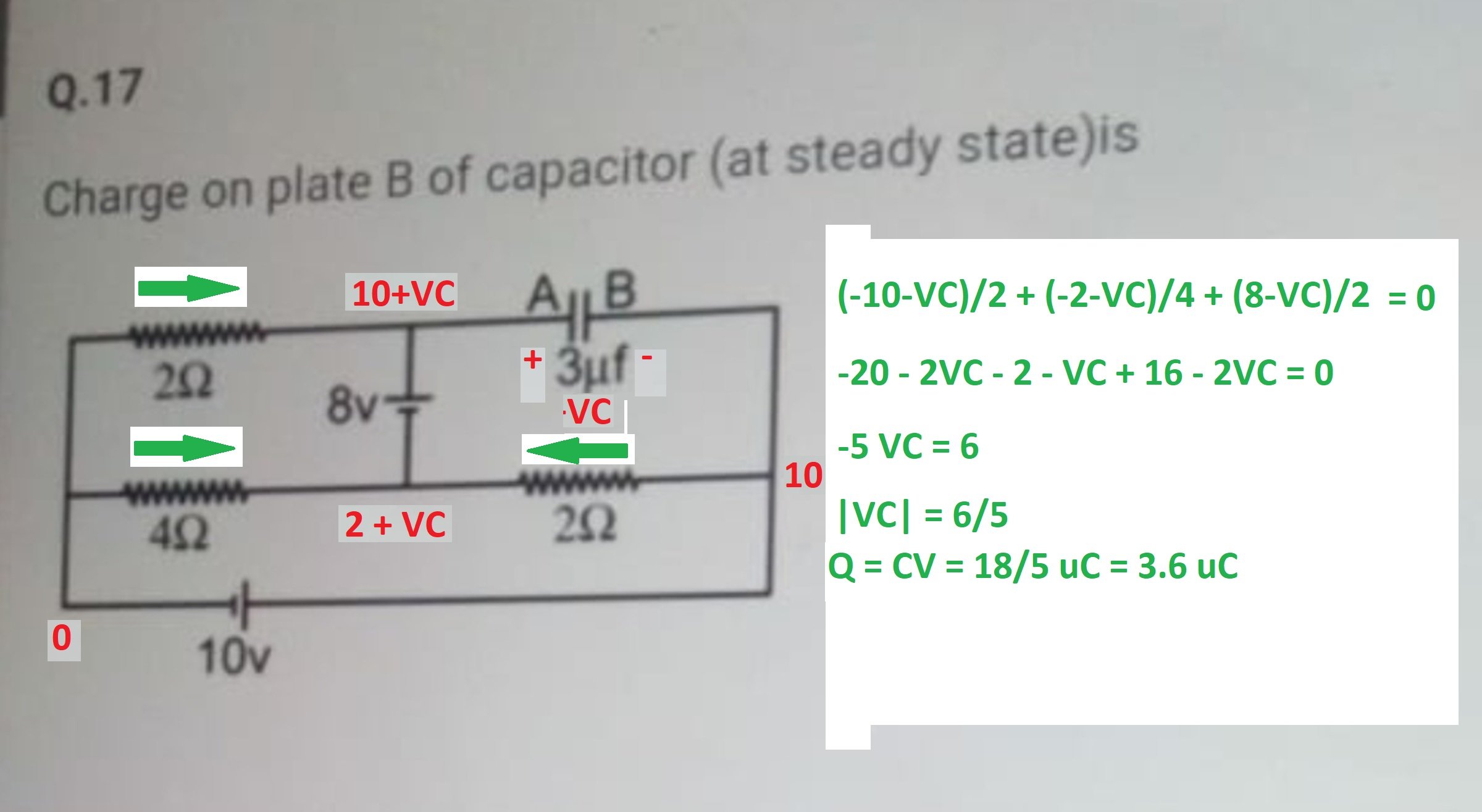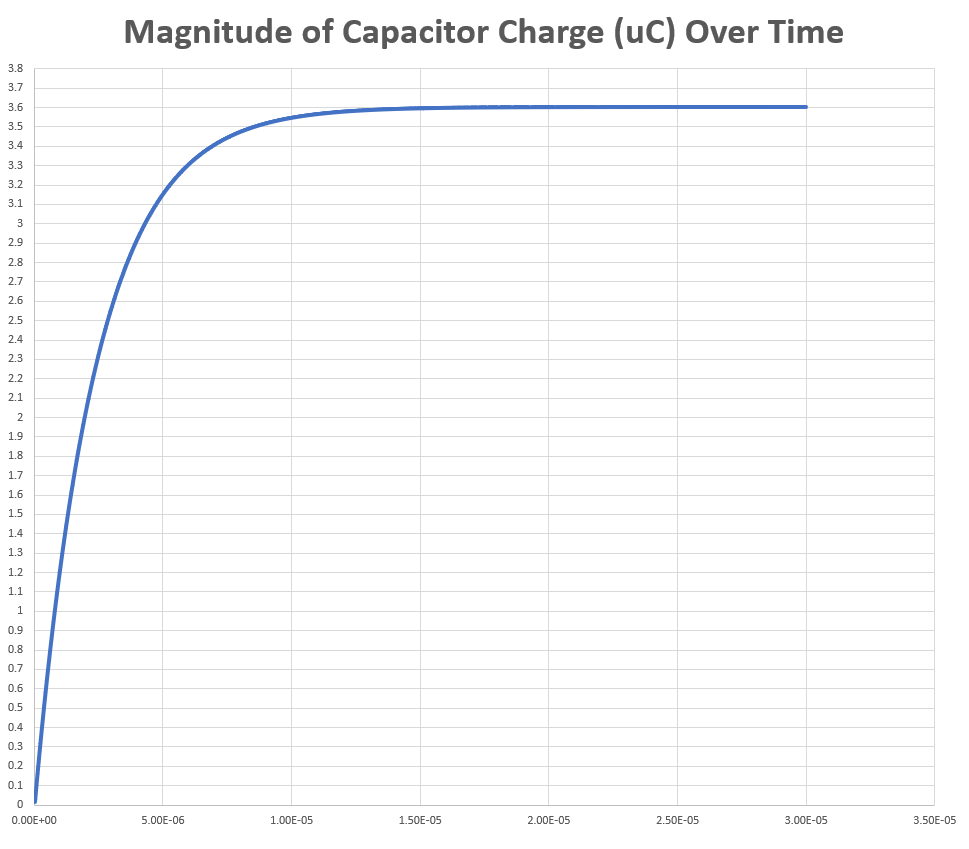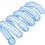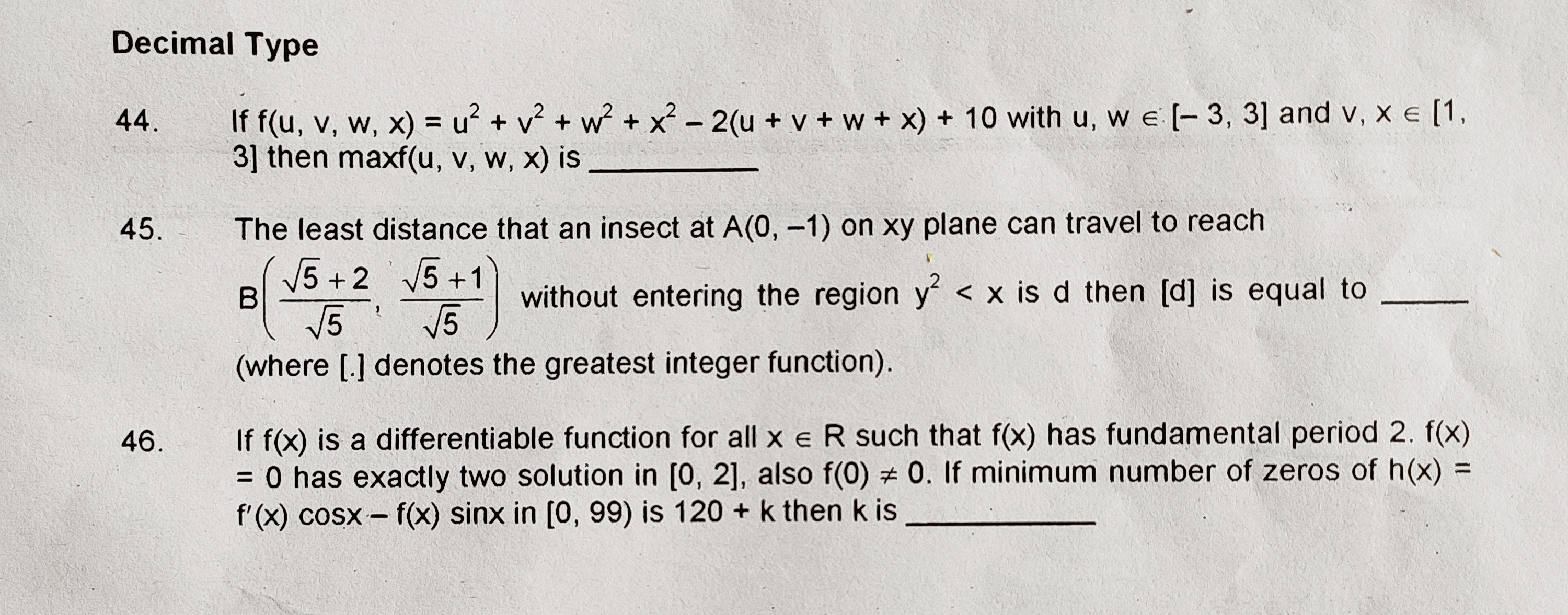# Capacitor Charge Problem

Here is a question shared by Neeraj.I have included in the image the analysis necessary to determine the steady state capacitor charge magnitude. The Python code below uses numerical integration to compute the evolution of the capacitor charge over time. Within about 20 microseconds, the code solution converges to the known steady state solution.1 2 3 4 5 6 7 8 9 10 11 12 13 14 15 16 17 18 19 20 21 22 23 24 25 26 27 28 29 30 31 32 33 34 35 36 37 38 39 40 41 42 43 44 import math dt = 10.0**(-9.0) u = 10.0**(-6.0) C = 3.0*u ###################################### t = 0.0 count = 0 VC = 0.0 I1 = (0.0 - (10.0+VC))/2.0 I2 = (0.0 - (2.0+VC))/4.0 I3 = (10.0 - (2.0+VC))/2.0 IC = I1 + I2 + I3 # IC = C*VCd VCd = IC/C while t <= 30.0*u: VC = VC + VCd*dt I1 = (0.0 - (10.0+VC))/2.0 I2 = (0.0 - (2.0+VC))/4.0 I3 = (10.0 - (2.0+VC))/2.0 IC = I1 + I2 + I3 # IC = C*VCd VCd = IC/C Q = C*VC t = t + dt count = count + 1 if count%10 == 0: print t,(abs(Q)/u)Note by Steven Chase
11 months, 4 weeks ago

This discussion board is a place to discuss our Daily Challenges and the math and science related to those challenges. Explanations are more than just a solution — they should explain the steps and thinking strategies that you used to obtain the solution. Comments should further the discussion of math and science.

When posting on Brilliant:

• Use the emojis to react to an explanation, whether you're congratulating a job well done , or just really confused .
• Ask specific questions about the challenge or the steps in somebody's explanation. Well-posed questions can add a lot to the discussion, but posting "I don't understand!" doesn't help anyone.
• Try to contribute something new to the discussion, whether it is an extension, generalization or other idea related to the challenge.

MarkdownAppears as
*italics* or _italics_ italics
**bold** or __bold__ bold
- bulleted- list
• bulleted
• list
1. numbered2. list
1. numbered
2. list
Note: you must add a full line of space before and after lists for them to show up correctly
paragraph 1paragraph 2

paragraph 1

paragraph 2

[example link](https://brilliant.org)example link
> This is a quote
This is a quote
    # I indented these lines
# 4 spaces, and now they show
# up as a code block.

print "hello world"
# I indented these lines
# 4 spaces, and now they show
# up as a code block.

print "hello world"
MathAppears as
Remember to wrap math in $$ ... $$ or $ ... $ to ensure proper formatting.
2 \times 3 $2 \times 3$
2^{34} $2^{34}$
a_{i-1} $a_{i-1}$
\frac{2}{3} $\frac{2}{3}$
\sqrt{2} $\sqrt{2}$
\sum_{i=1}^3 $\sum_{i=1}^3$
\sin \theta $\sin \theta$
\boxed{123} $\boxed{123}$

Sort by:

- 11 months, 4 weeks ago

Yeah Very nice, I wanted this method only. Maybe this is long but its crazy.
Thank you so much.

- 11 months, 4 weeks ago

You're welcome. What is long?

- 11 months, 4 weeks ago

@Steven Chase The code is long for me. Maybe for you not because you are a experienced LPE.
Thanks again .

- 11 months, 4 weeks ago

Yeah, I think it took me about 3 minutes

- 11 months, 4 weeks ago

@Steven Chase it will take me minimum 10 min to type this code. You are a legend ,smart and stud.

- 11 months, 4 weeks ago

@Steven Chase try the 45th problem- 11 months, 2 weeks ago